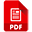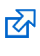# That Blue Square Thing

## AQA Computer Science GCSE

This page is up to date for the new AQA 8525 syllabus for the 2022 exam.

### Data Representation - Hexadecimal Numbers

Hexadecimal numbers use base 16 - so you can use one digit to county up to 15. The letters A to F are added on after 9, so A = 10, B = 11 and so on until F = 15. That gives you 16 possible values - including the 0.

Hexadecimal isn't used by computers, but it is an easy thing for humans to use when we're trying to talk in binary. And it converts really easily.

Just remember that A = 10.Hexadecimal basics - notes on how it all works.The 16 times table - and how not to panic when doing the maths.

One really important thing to remember is that the hexadecimal number FF = 255 - which is the same as the 8 bit binary number 11111111. So, 255 is the largest number which can be represented using 8 bit binary (a byte) and a 2 digit hexadecimal number.

Just remember that A = 10 and F = 15. It's really easy to get into thinking that A is 11 and F is 16. It's not! Get this right and hexadecimal is a piece of cake.

You need to be able to convert from:

• hexadecimal to 8 bit binary
• 8 bit binary to hexadecimalConverting Hex to Binary/Binary to Hex - the methodsQuestions: Hex to Binary/Binary to Hex (answers)

Converting to and from decimal is harder than to and from binary...Converting Hex to Decimal/Decimal to Hex - the methodsQuestions: Hex to Decimal/Decimal to Hex (answers)

Here are some photos taken in class which summarise the methods:

Binary to Hex/Hex to Binary

Decimal to Hex/Hex to Decimal

#### Revision

There's likely to be one or two marks on hexadecimal on the exam.Hexadecimal questions - some hex conversion questionsAnswers - don't cheat, try and work things out first

The BBC Bitesize website has a section on hexadecimalthat you might want to look at. Well worth a look.